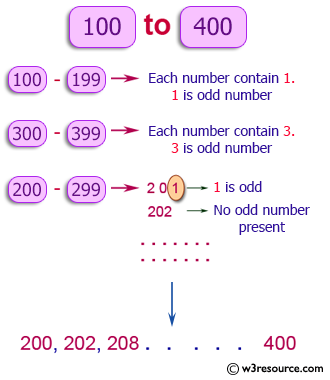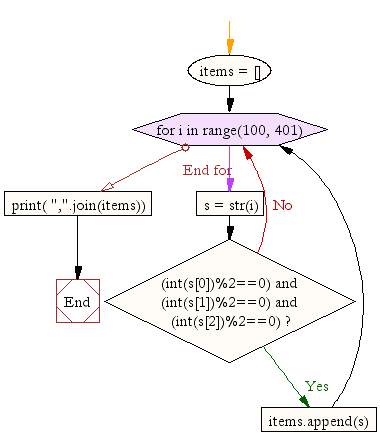﻿ Python Exercise: Find numbers between two numbers where each digit of a number is an even number - w3resource# Python Exercise: Find numbers between two numbers where each digit of a number is an even number

## Python Conditional: Exercise - 16 with Solution

Write a Python program to find numbers between 100 and 400 (both included) where each digit of a number is an even number. The numbers obtained should be printed in a comma-separated sequence.

Pictorial Presentation:Sample Solution:

Python Code:

``````items = []
for i in range(100, 401):
s = str(i)
if (int(s)%2==0) and (int(s)%2==0) and (int(s)%2==0):
items.append(s)
print( ",".join(items))
```
```

Sample Output:

```200,202,204,206,208,220,222,224,226,228,240,242,244,246,248,260,262,264,266,268,280,282,284,286,288,400
```

Flowchart:## Visualize Python code execution:

The following tool visualize what the computer is doing step-by-step as it executes the said program:

Python Code Editor:

Have another way to solve this solution? Contribute your code (and comments) through Disqus.

What is the difficulty level of this exercise?

Test your Python skills with w3resource's quiz

﻿

## Python: Tips of the Day

Returns a list with n elements removed from the beginning

Example:

```def tips_take(itr, n = 1):
return itr[:n]
print(tips_take([1, 2, 3], 5))
print(tips_take([1, 2, 3], 0))
```

Output:

```[1, 2, 3]
[]
```